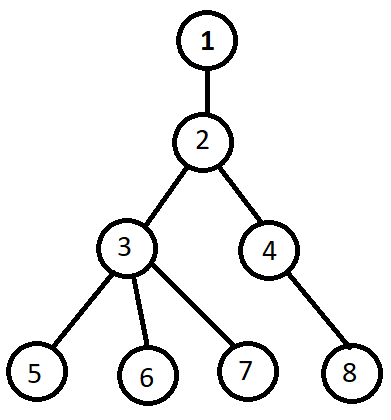# Simple Andfire_tornadoReplay of National High S...
Limits 2s, 512 MB

You are given a tree containing $N$ nodes. The nodes are numbered from $1$ to $N$. The root node of that tree is $R$. Every node in that tree is associated with a value. Let, $X_u$ be the value associated with node $u$.

You have to answer $Q$ queries. In each query, you will be given a pair of integers $(u, k)$ and you have to calculate the Bitwise AND of all the values of $k$-th child in the subtree of node $u$. If there is no $k$-th child in subtree then the answer will be $-1$.

## Input

First line of the Input will have a single integer $T$ ($1 \leq T \leq 5$) denoting the number of test cases.

The first line of every test case contains three integers $N$ ($1 \leq N \leq 10^5$), $Q$ ($1 \leq Q \leq 10^5$), $R$ ($1 \leq R \leq N$) representing the number of nodes in the tree, number of queries, and the root node of the tree respectively.

Next line will contain $N$ space-separated integers representing the values of $X_u$ ($0 \leq X_u \leq 10^9$).

Next $N-1$ lines will contain a pair of integers $(u, v)$ which denotes there is an edge between node $u$ and node $v$. Here, ($1 \leq u, v \leq N$).

Next $Q$ line will have a pair of integers $(u, k)$ representing each query. Here, ($1 \leq u \leq N$) and ($0 \leq k \leq 10^5$).

$1 \leq T \leq 5$, $1 \leq N \leq 10^4$, $1 \leq Q \leq 10^4$, $0 \leq X_u \leq 10^9$, $0 \leq k \leq10^5$

$1 \leq T \leq 5$, $1 \leq N \leq 10^5$, $1 \leq Q \leq 10^5$, $0 \leq X_u \leq 10^9$, $0 \leq k \leq 1$

Original constraints

## Output

For each query, you have to print the answer of that query in a new line.

## Sample

InputOutput
1
8 6 1
3 23 5 6 15 11 14 10
1 2
2 4
4 8
3 5
3 6
3 7
3 2
2 2
3 1
4 2
1 2
1 3
1 1

10
10
-1
4
10
23Here, 0-th child of node $2$ is ${2}$. 1st children of node $2$ are ${3, 4}$. 2nd children of node $2$ are ${5, 6, 7, 8}$. And there is no 3rd child of node $2$.DFSuDebug

### Submit